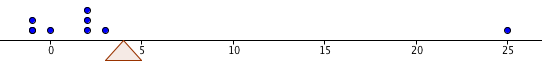﻿ Mean, Median, and Mode
MEAN, MEDIAN, and MODE
by Dr. Carol JVF Burns (website creator)
Follow along with the highlighted text while you listen!

Statistics is the discipline devoted to organizing, summarizing, and drawing conclusions from data.

Given a collection of data, it is often convenient to come up with a single number that somehow describes its center;
a number that in some way is representative of the entire collection.
Such a number is called a measure of central tendency.

The two most popular measures of central tendency are the mean and the median.
Another measure sometimes used to describe a ‘typical’ data value is the mode.

the MEAN of a data set

The mean (or average) is already familiar to you: add up the numbers, and divide by how many there are:

DEFINITION mean, average
The mean (or average) of the $\,n\,$ data values $$\,\cssId{s14}{x_1, x_2, x_3, \ldots, x_n}\,$$ is denoted by $\,\bar{x}\,$ (read as ‘$\,x\,$ bar’) and is given by the formula \begin{alignat}{2} \cssId{s19}{\bar{x}}\ &\cssId{s20}{= \frac{x_1 + x_2 + x_3 + \cdots + x_n}{n}}\\ &\cssId{s21}{= \frac{\sum_{i=1}^n\ x_i}{n}}\ \ &&\cssId{s22}{\text{(using summation notation)}}\\ &\cssId{s23}{= \frac1n\sum_{i=1}^n\ x_i}\ \ &&\cssId{s24}{\text{(an alternate version of summation notation)}}\\ \end{alignat} Thus, to find the mean of $\,n\,$ data values, you add them up and then divide by $\,n\,$.

Similarly, the mean of the $\,n\,$ data values   $\,y_1, y_2, y_3, \ldots, y_n\,$
would be denoted by $\,\bar{y}\,$ and read as ‘$\,y\,$ bar’.

Since dividing by $\,n\,$ is the same as multiplying by $\,\frac{1}{n}\,$,
the notation $\displaystyle\,\frac{\sum_{i=1}^n\ x_i}{n}\,$ is more commonly written as   $\,\frac 1n\sum_{i=1}^n\ x_i\,$   or   $\displaystyle\,\frac 1n\sum_{i=1}^n\ x_i\,$  .

EXAMPLE:
Find the mean of these data values:   $2,\ -1,\ 2,\ 3,\ 0,\ 25,\ -1,\ 2$
There are $\,8\,$ data values.
The mean is found by adding them up and then dividing by $\,8\,$: $$\cssId{s42}{\frac{2+(-1)+2+3+0+25+(-1)+2}{8}} \cssId{s43}{= \frac{32}{8}} \cssId{s44}{= 4}$$ As discussed in Average of Three Signed Numbers,
the mean gives the balancing point for the distribution, in the following sense:
if eight pebbles of equal weight are placed on a ‘number line see-saw’:
two pebbles at $\,-1\,$, one pebble at $\,0\,$, three pebbles at $\,2\,$, one pebble at $\,3\,$, and one pebble at $\,25\,$;
then the support would have to be placed at $\,4\,$ for the see-saw to balance perfectly!Notice in the previous example that the number $\,25\,$ seems to be unusually large, compared to the other numbers.
An outlier is an unusually large or small observation in a data set.
A drawback of the mean is that its value can be greatly affected by the presence of even a single outlier.
If the outlier $\,25\,$ is changed to $\,250\,$, then the new mean would be $\,32.125\,$,
which does not seem at all representative of a ‘typical’ number in this data set!

the MEDIAN of a data set

The median, on the other hand, is quite insensitive to outliers.

Just as the median strip of a highway goes right down the middle,
the median of a set of numbers goes right through the middle of the ordered list.
Of course, only lists with an odd number of values have a true middle:
the middle number in the ordered list $\,5,\ 7,\ 20\,$ is $\,7\,$.
See how the definition below solves the problem when there are an even number of data values:

DEFINITION median
To find the median of a set of $\,n\,$ data values,
first order the observations from least to greatest (or greatest to least).

If $\,n\,$ is odd, then the median is the number in the exact middle of the list.
That is, the median is the data value in position $\,\frac{n+1}{2}\,$ of the ordered list.

If $\,n\,$ is even, then the median is the average of the two middle members of the ordered list.
That is, the median is the average of the data values in positions $\,\frac{n}{2}\,$ and $\,\frac{n}{2}+1\,$
of the ordered list.
EXAMPLE:
Question:
Find the median of these data values:   $\,2,\ -1,\ 2,\ 3,\ 0,\ 25,\ -1,\ 2$
(This is the same data set as in the previous example.)
Solution:
Begin by ordering the eight data values from least to greatest: $$\cssId{s84}{\underset{\text{position 1}}{\underset{\uparrow}{-1,\strut}}}\ \ \ \ \cssId{s85}{\underset{\text{position 2}}{\underset{\uparrow}{-1,\strut}}}\ \ \ \ \cssId{s86}{\underset{\text{position 3}}{\underset{\uparrow}{0,\strut}}}\ \ \ \ \cssId{s87}{\overset{\text{the two ‘middle’ members}} {\ \ \overbrace{ \underset{\text{position 4}}{\underset{\uparrow}{2,\strut}}\ \ \ \ \underset{\text{position 5}}{\underset{\uparrow}{2,\strut}} }}}\ \ \ \ \cssId{s88}{\underset{\text{position 6}}{\underset{\uparrow}{2,\strut}}}\ \ \ \ \cssId{s89}{\underset{\text{position 7}}{\underset{\uparrow}{3,\strut}}}\ \ \ \ \cssId{s90}{\underset{\text{position 8}}{\underset{\uparrow}{25\strut}}}$$ There are an even number of values, so we average the values in positions four and five:
the median is $\,\frac{2+2}{2} = 2\,$.

Note that, for this data set, the median seems to do a better job than the mean in representing a ‘typical’ member.
Note also that if the outlier $\,25\,$ is changed to $\,250\,$, it doesn't affect the median at all!
the MODE of a data set

Finally, a mode is a value that occurs ‘most often’ in a data set.
Whereas a data set has exactly one mean and median, it can have one or more modes.

For example, consider these data values:   $\,2,\ -1,\ 2,\ 3,\ 0,\ 25,\ -1,\ 2\,$
Re-group them occurring to their frequency: \begin{align} \cssId{s102}{2,\ \ 2,\ \ 2,}\ \ \ \ &\cssId{s103}{\text{three occurrences of the number 2}}\\ \cssId{s104}{-1,\ \ -1,}\ \ \ \ &\cssId{s105}{\text{two occurrences of the number -1}}\\ \cssId{s106}{0,}\ \ \ \ &\cssId{s107}{\text{one occurrence of the number 0}}\\ \cssId{s108}{3,}\ \ \ \ &\cssId{s109}{\text{one occurrence of the number 3}}\\ \cssId{s110}{25}\ \ \ \ &\cssId{s111}{\text{one occurrence of the number 25}} \end{align} The mode of this data set is $\,2\,$, since this data value occurs three times, and this is the most occurrences of any data value.

Every member of the data set   $\,3,\ 7,\ 9\,$   is a mode, since each value occurs only once.

The data set   $\,3,\ 3,\ 7,\ 7,\ 9\,$   has two modes: $\,3\,$ and $\,7\,$.
Each of these numbers occurs twice, and no number occurs more than two times.

Master the ideas from this section